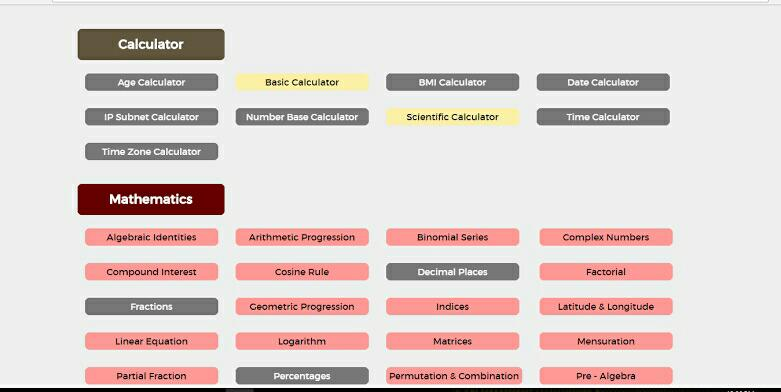# Why Should Everyone Use Nickzom Calculator?

Nickzom CalculatorThe Nickzom Calculator has been in existence since 2013, launched on the 2nd of December, and has had major developments in its functionality till date with the Pro version Nickzom Calculator+ coming in place. This app is user friendly, easy navigation and requires no Internet connection after installation. Not enough, there’s more.

The Nickzom Calculator is a web, mobile and desktop platform that solves calculation problems and display the steps of the solution right to the final answer.

Now imagine you have a calculation to solve and you do not know how to go about it or you have consulted your textbook to see how various questions are solved and you’re utterly disappointed because, they solved only the simpler ones, or you don’t just get how the problem was solved. It is because of situations like this that Nickzom Calculator exists. This app solves calculations on Mathematics, Physics, Engineering, Chemistry, Finance, Accounting, Geology, Economics, Unit Conversions etcetera for quick and easy understanding.

The goal of this math app is to provide one with solutions and steps to his or her calculations, alongside a basic and scientific calculator, making that topic or assignment less complex.

Nickzom Calculator covers over 50,000 calculations in over 12 different fields. Users can click on topics of interest, input their parameters, and hit Calculate. Nickzom Calculator immediately displays the steps on how the problem was solved till the final answer, with appropriate explanations at each point. This app presents the formula, equations, parameters and operations, steps and answer to your question.It comes with a sub-platform that allows secondary and tertiary students to post and answer assignments. It also has an instant messaging feature that enables users to communicate with each other. Cool right!

You can also reach out to the developers by clicking the “Let us help you solve that maths” banner to solve Maths problems not readily available on the platform.

While Nickzom calculator seems tailored to meet the needs of students within a learning environment, it is also important to point out that its usefulness extends beyond that.

In Engineering, it covers:

Economics (Economic Equivalence and Depreciation), Basic Electrical, Basic Electronics, Calculus (Differentiation and Integration), Laplace Transform, Linear Algebra (Matrix Arithmetic, Solutions of Linear Systems), Linear Programming (Without Artificial Variables), Mechanics (Statics and Dynamics) and more.

In Mathematics, it covers:

Algebraic Identities, Arithmetic Progression (Sequences and Series), Binomial Series, Complex Numbers, Compound Interest, Cosine Rule, Factorial, Geometric Progression (Sequences, Series and Infinite Series), Indices, Latitude and Longitude, Linear Equation, Logarithm and lots more.

So much in-depth knowledge and explanations can be gotten from the various Math laws and theorems available.

In Physics, topics like:

Elasticity, Electric Field, Electrolysis, Electro magnetism, Energy Quantization, Equilibrium Of Forces, Gas Laws, Gravitational Field, Heat Energy, Machines, Magnetic Field, Motion, Pressure, Projectile, Radioactivity, Simple A.C. Circuit are covered and more.

In Unit Conversions, Nickzom Calculator converts the following units:

Temperature Units,

Mathematical Number Bases (Binary, Decimal, Hexadecimal, Octal and Custom [3 – 9]), Coordinates Units (Cylindrical, Spherical, Rectangular or Cartesian and Polar).

If you are just looking for a simple scientific calculator, this app also works well too. Everyone can easily use this app.

The Pro version Nickzom Calculator+ has 30+ unit conversions and 30+ Chemistry calculation solutions, which is updated on a regular basis with more content and solutions to calculation problems. Though, will cost you \$25.99, still worth every penny.

The Nickzom Calculator is one of the best mathematical problem solver applications, with over 10,000 installations on Google play store, and over 180 reviews from various users, with a 4.7 star rating.

Math tutorials and mathematical lessons in class or study groups can also be carried out using this Calculator.

If you are a Math student, Lecturer, Researcher or have any thing to do with Mathematics, all you need to do is to go to the site below, download the desktop or mobile app, click on the title of the topic and navigate your way through. Don’t forget to rate this app and also support by buying the professional version.

One can access Nickzom Calculator via any of these channels:

## One thought on “Why Should Everyone Use Nickzom Calculator?”

1.Market Strom αθηνα says:

I’m not sure where you are getting your information, but great topic.
I needs to spend some time learning much more or understanding more.
Thanks for fantastic information I was looking for this information for my mission.